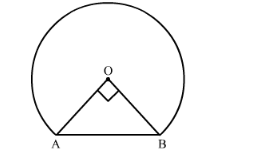# In the following figure, shows the cross-section of railway tunnel.

Question:

In the following figure, shows the cross-section of railway tunnel. The radius OA of the circular part is 2 m. If ∠AOB = 90°, calculate:

(i) the height of the tunnel

(ii) the perimeter of the cross-section

(iii) the area of the cross-section.Solution:

We have a cross section of a railway tunnel.is a right angled isosceles triangle, right angled at O. let OM be perpendicular to AB.

(i) We have to find the height of the tunnel. We have,

$\mathrm{OA}=2 \mathrm{~m}$

Use Pythagoras theorem in $\triangle \mathrm{OAB}$ to get,

$\mathrm{AB}=\left(\sqrt{2^{2}+2^{2}}\right) \mathrm{m}$

$=2 \sqrt{2} \mathrm{~m}$

Let the height of the tunnel be h. So,

Area of $\Delta \mathrm{OAB}=\frac{1}{2}(2)(2)$

$\frac{1}{2}(2 \sqrt{2})(\mathrm{OM})=2$

Thus,

$\mathrm{OM}=\sqrt{2} \mathrm{~m}$

Therefore,

$h=(2+\sqrt{2}) \mathrm{m}$

(ii)

Perimeter of cross-section is,

$=$ Major are $\mathrm{AB}+\mathrm{AB}$

$=2(\pi)(2)\left(\frac{3}{4}\right)+2 \sqrt{2}$

$=(3 \pi+2 \sqrt{2}) m$

(iii)

Area of cross section,

$=\frac{3}{4}($ Area of circle $)+$ Area of $\triangle \mathrm{OAB}$

$=(\pi)(2)^{2}\left(\frac{3}{4}\right)+\frac{1}{2}(2)(2)$

$(3 \pi+2) m^{2}$